Want to share your content on R-bloggers? click here if you have a blog, or here if you don't.

### Introduction

This is the 19th post in the series Elegant Data Visualization with ggplot2. In the previous post, we learnt to modify the title, label and bar of a legend. In this post, we will learn about faceting i.e. combining plots.

### Libraries, Code & Data

We will use the following libraries in this post:

All the data sets used in this post can be found here and code can be downloaded from here.

### Faceting – Intro

Let us continue with the scatter plot examining the relationship between displacement and miles per gallon but let us make one additional change. We now want 3 sub plots for each type of cylinder. How can we do this? We can split or group the data by cylinder type and plot the subset of data which means dealing with 3 different data sets, plotting 3 plots and arranging them for comparison. ggplot2 offers the following 2 functions which allow us to plot subset of data with a simple formula based interface:

• `facet_grid()`
• `facet_wrap()`

Faceting allows us to create multiple sub plots. It partitions a plot into a matrix of panels with each panel showing a different subset of data.

### Vertical

`facet_grid()` allows us to split up the data by one or two discrete variables and create sub plots. The sub plots can be arranged horizontally or vertically using a formula of the form `vertical ~ horizontal`. In the below example, 3 sub plots are created, one each for the levels of the `cyl` variable and the sub plots are arranged vertically

```ggplot(mtcars, aes(disp, mpg)) +
geom_point() +
facet_grid(cyl ~ .)```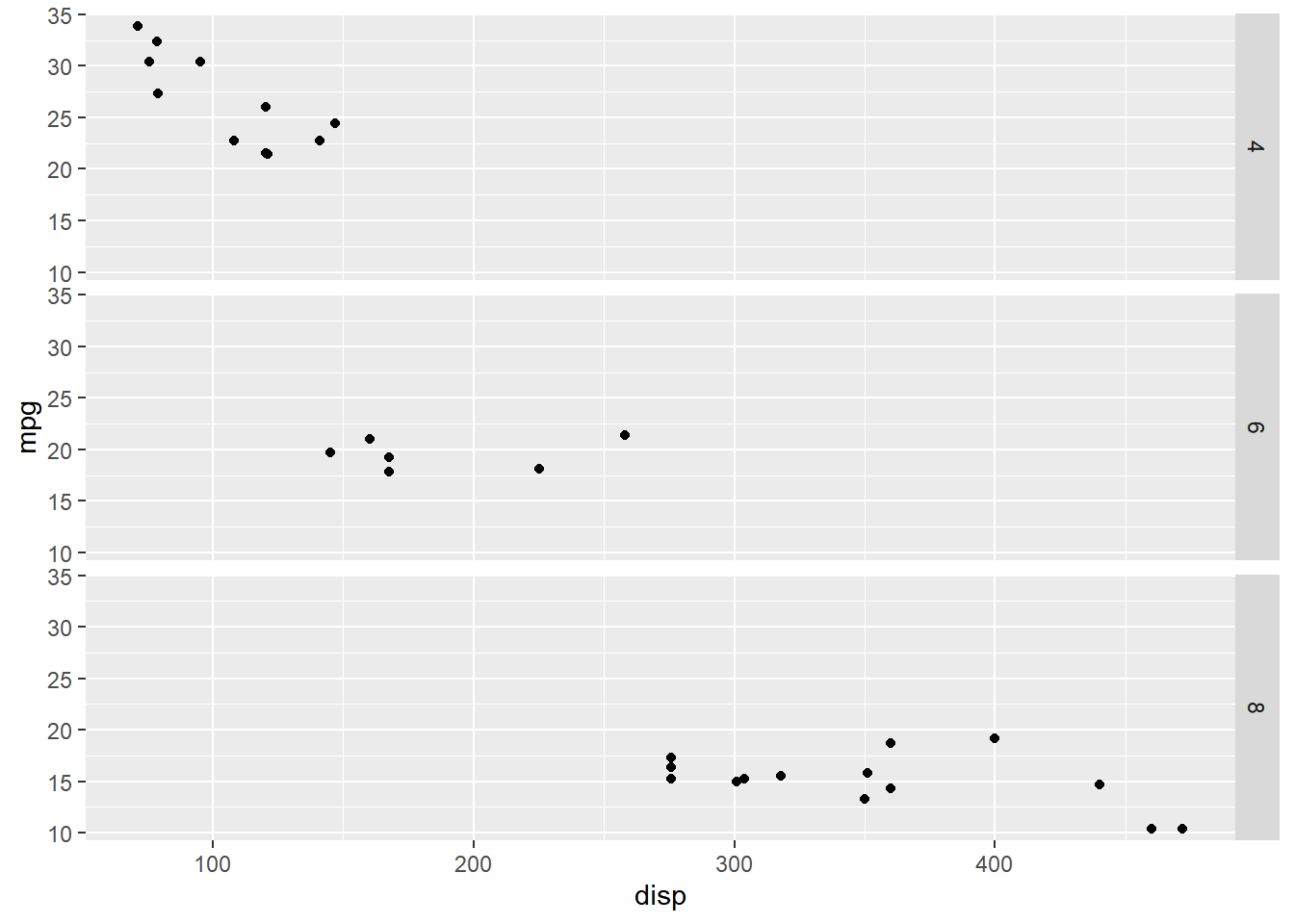### Horizontal

Below we reproduce the previous example but arrange the sub plots horizontally.

```ggplot(mtcars, aes(disp, mpg)) +
geom_point() +
facet_grid(. ~ cyl)```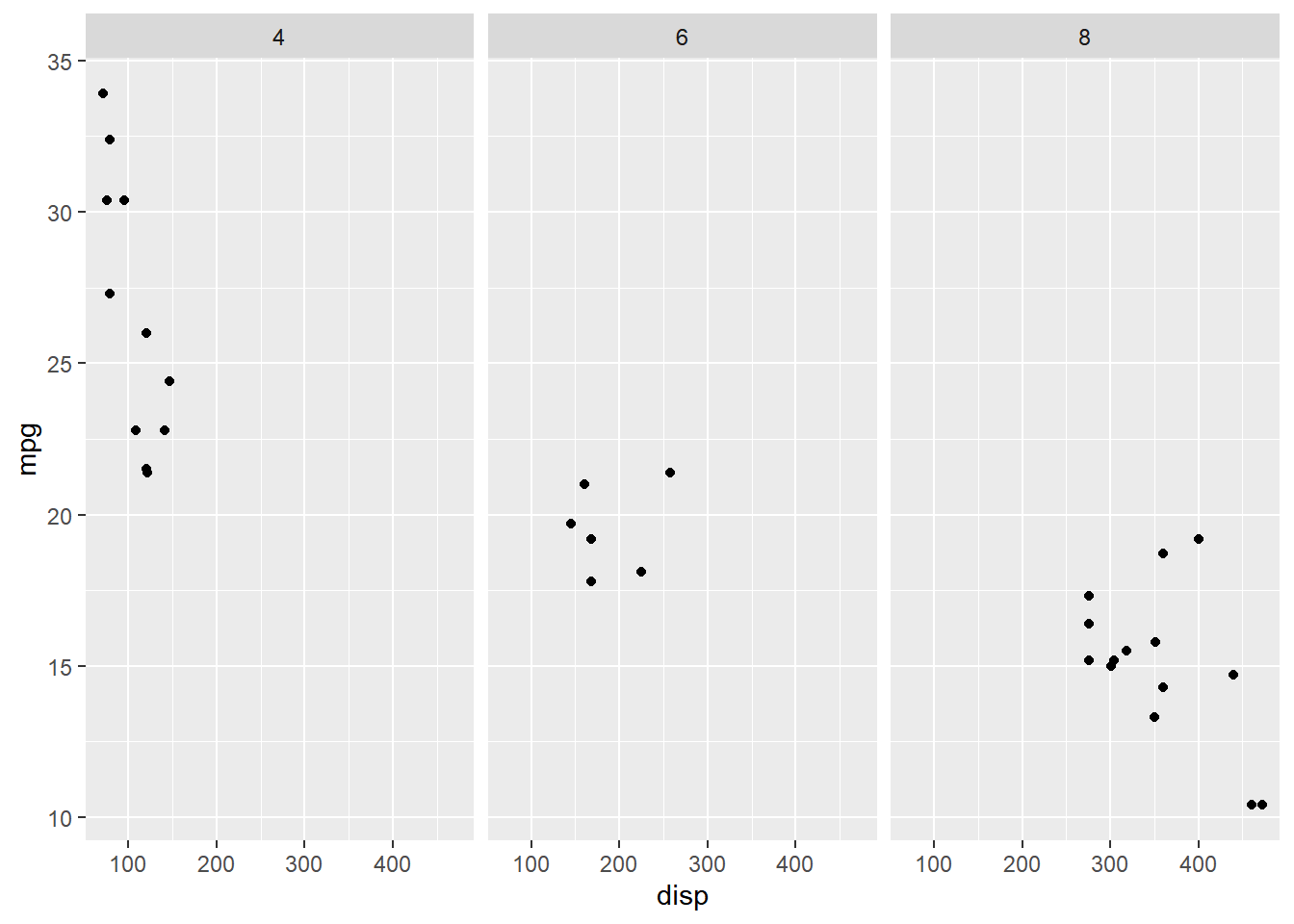### Vertical & Horizontal

In certain cases, we might want different discrete variables to represent the horizontal and vertical direction. In the below example, we examine the relationship between displacement and miles per gallon for different combinations of `cyl` and `gear` variables.

```ggplot(mtcars, aes(disp, mpg)) +
geom_point() +
facet_grid(cyl ~ gear)```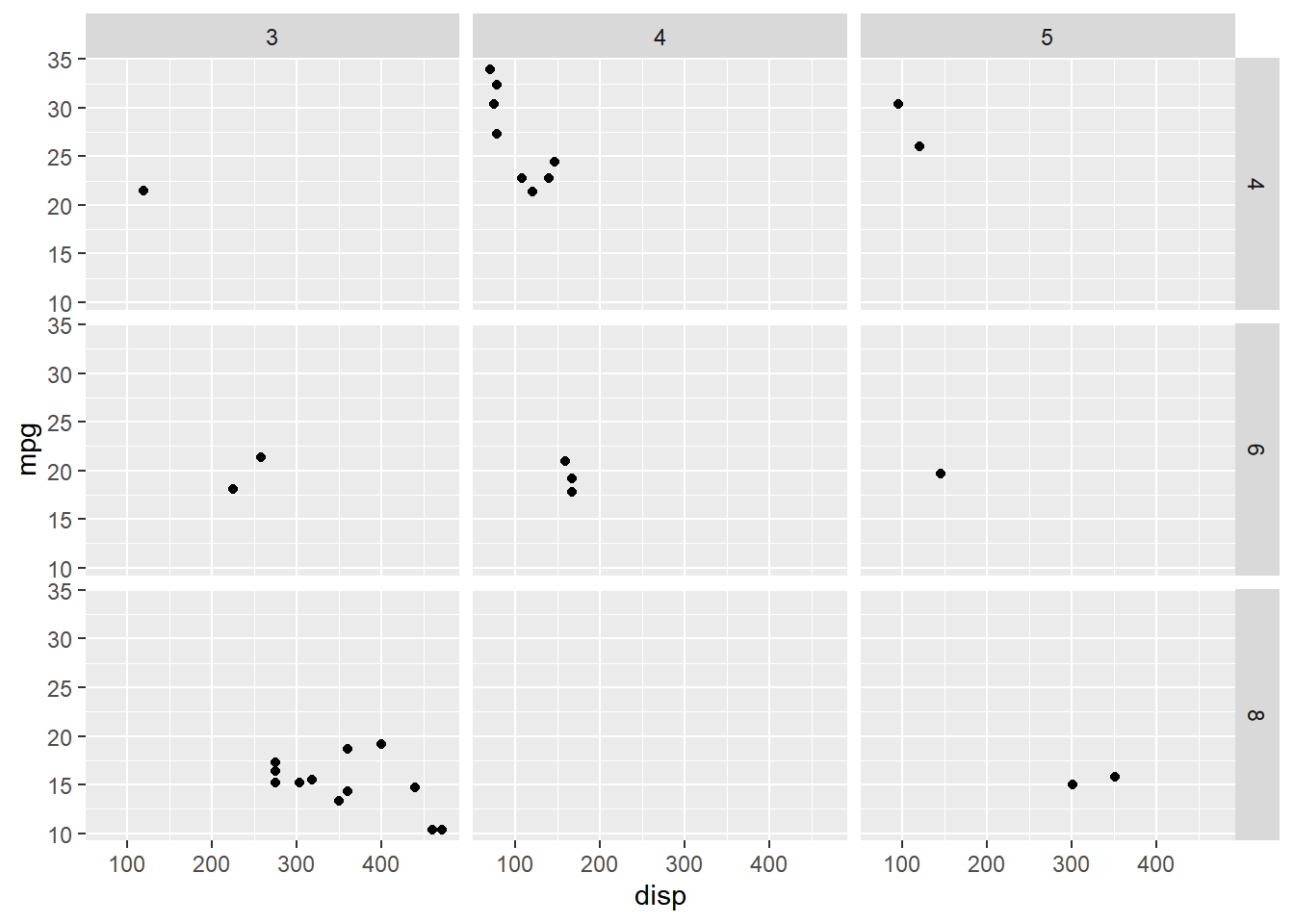Below, we switch the variables representing the vertical and horizontal directions.

```ggplot(mtcars, aes(disp, mpg)) +
geom_point() +
facet_grid(gear ~ cyl)```### Scales

If you carefully observe the second example, the range of `X` axis is same for all the 3 sub plots i.e. it is a fixed range. You can allow each of the sub plots to have different range using the `scales` argument and supplying it the value `'free'`.

```ggplot(mtcars, aes(disp, mpg, color = factor(cyl))) +
geom_point() +
facet_grid(. ~ cyl, scales = "free")```Now, each of the sub plot has a different range.

### Switch Labels

In the third example, the labels are displayed at the bottom for `X` axis and at the right for the `Y` axis. It can be changed using the `switch` argument and supplying the value `'both'`. The labels will now be displayed at the top for the `X` axis and at left for the `Y` axis. If you just want to change the labels for a particular axis, use the values `x` and `y` for the `X` and `Y` axis respectively.

```ggplot(mtcars, aes(disp, mpg)) +
geom_point() +
facet_grid(cyl ~ gear, switch = "both") ```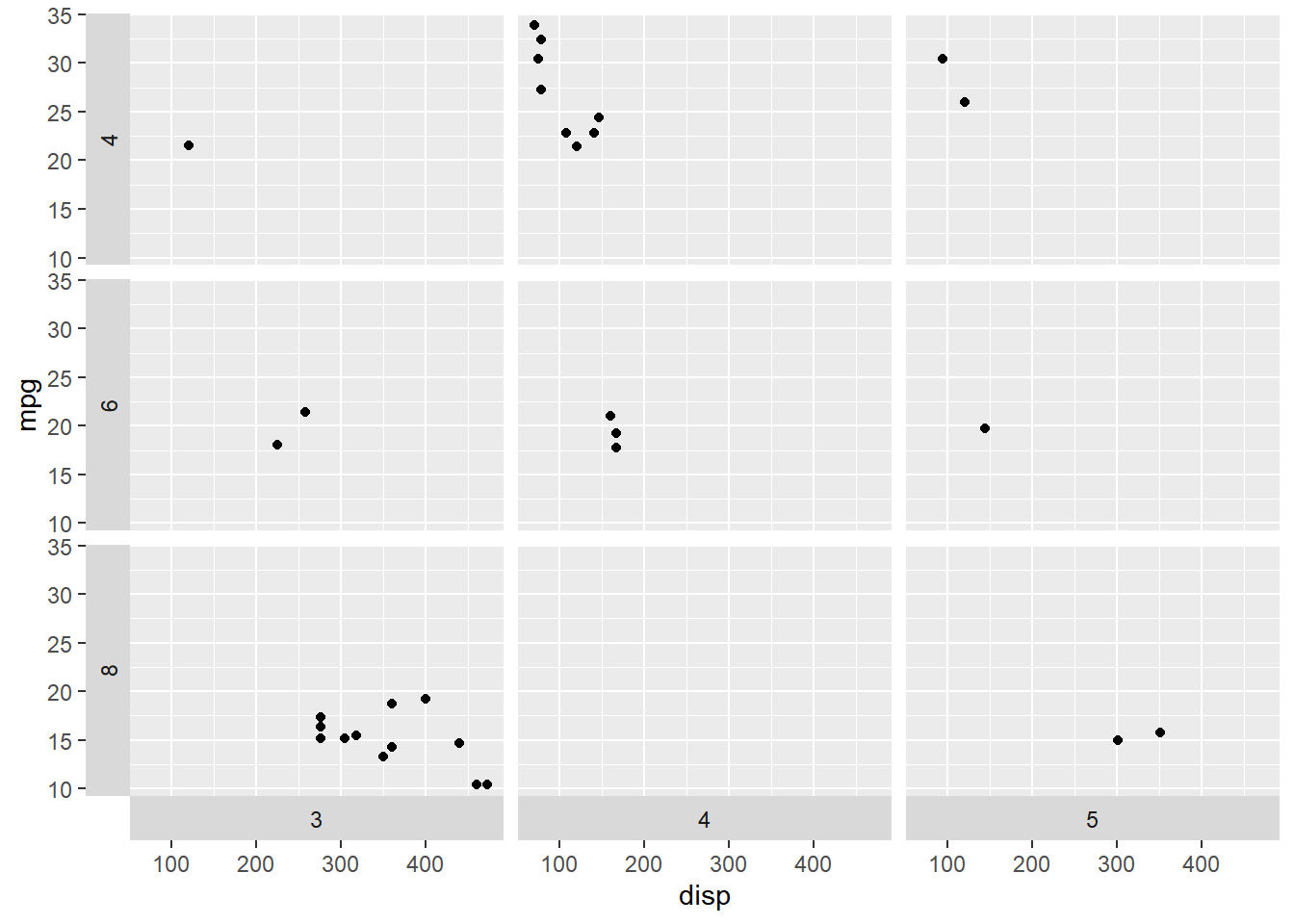### Wrap

`facet_wrap()` allows us to arrange sub plots in a certain number of rows and columns. In the below example, we will use `facet_wrap()` to arrange the sub plots in a single row.

```ggplot(mtcars, aes(disp, mpg)) +
geom_point() +
facet_wrap(~cyl)```### Specify Rows

To arrange the sub plots in a specific number of rows, use the `nrow` argument. In the below example, we arrange the sub plots in 2 rows.

```ggplot(mtcars, aes(disp, mpg)) +
geom_point() +
facet_wrap(~cyl, nrow = 2)```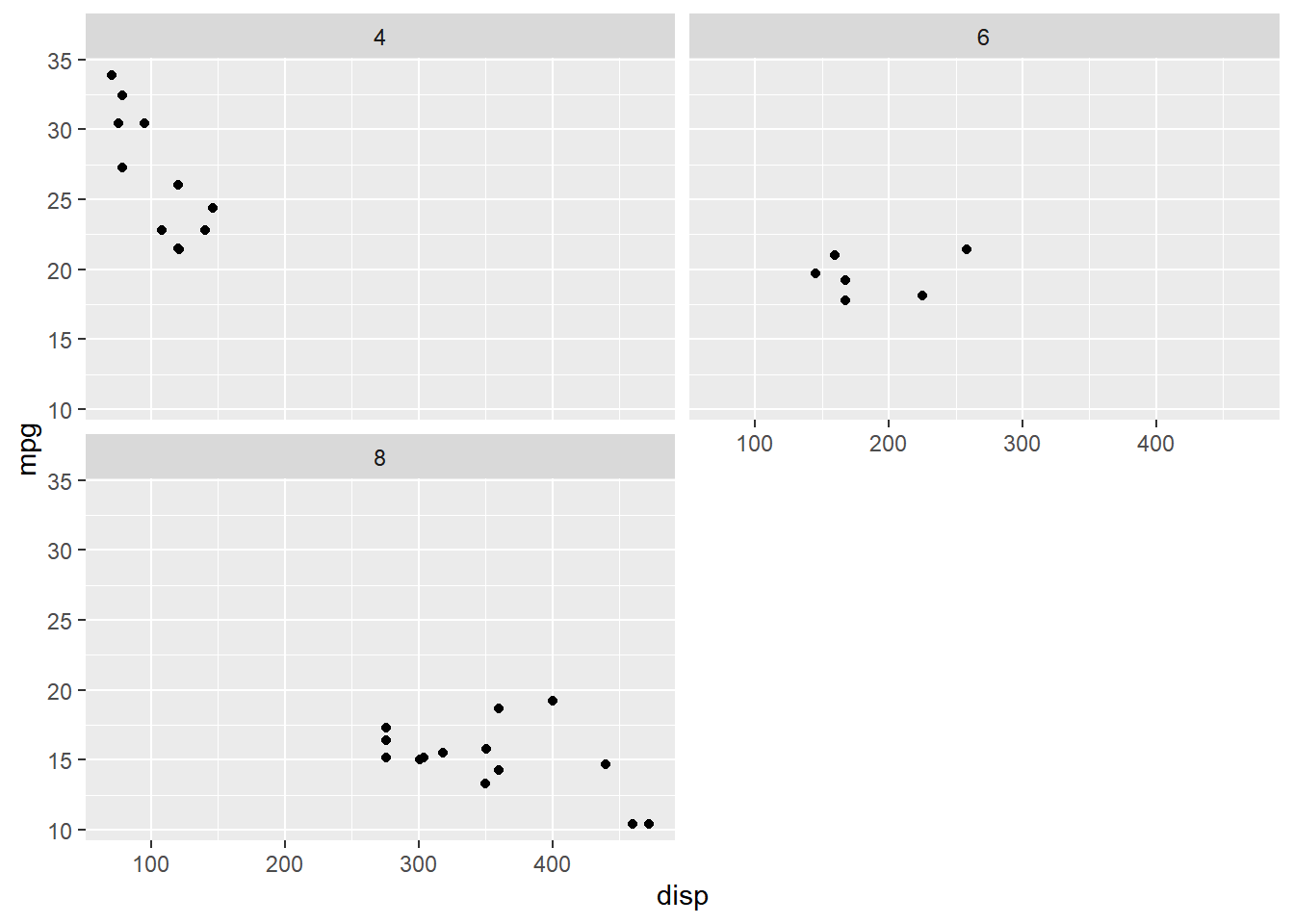### Specify Columns

Here, we arrange the sub plots in 3 columns instead of rows using the `ncol` argument.

```ggplot(mtcars, aes(disp, mpg)) +
geom_point() +
facet_wrap(~cyl, ncol = 3)```### Scales

You can allow each of the sub plots to have different range using the `scales` argument and supplying it the value `'free'`.

```ggplot(mtcars, aes(disp, mpg)) +
geom_point() +
facet_wrap(~cyl, scales = "free")```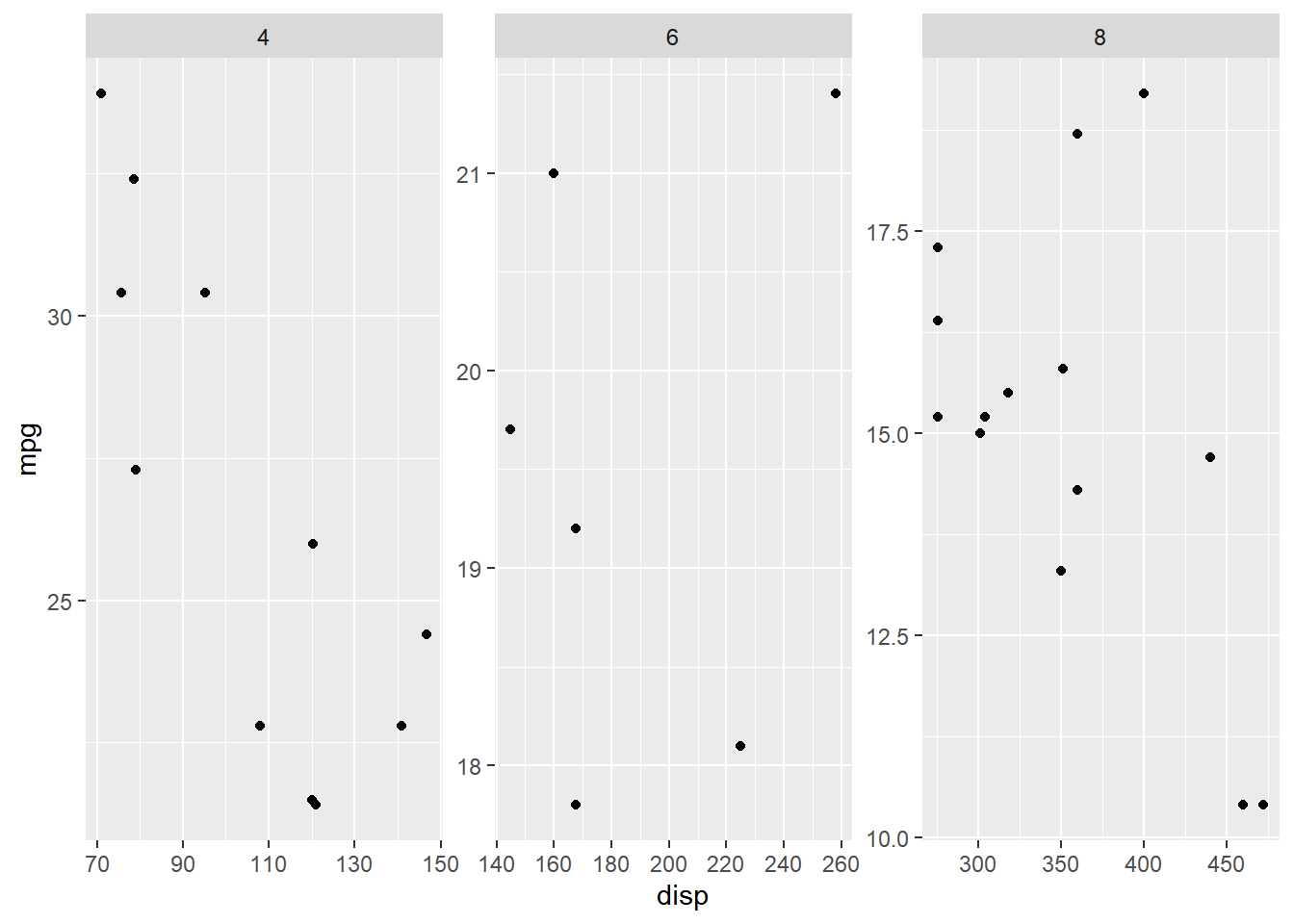### Rows & Columns

If 2 discrete variables are used to create the sub plots, we can either use the formula interface to specify the variables as shown below

```ggplot(mtcars, aes(disp, mpg)) +
geom_point() +
facet_wrap(~cyl + gear, nrow = 2)```or use a character vector of variable names.

```ggplot(mtcars, aes(disp, mpg)) +
geom_point() +
facet_wrap(c("cyl", "gear"), ncol = 2)```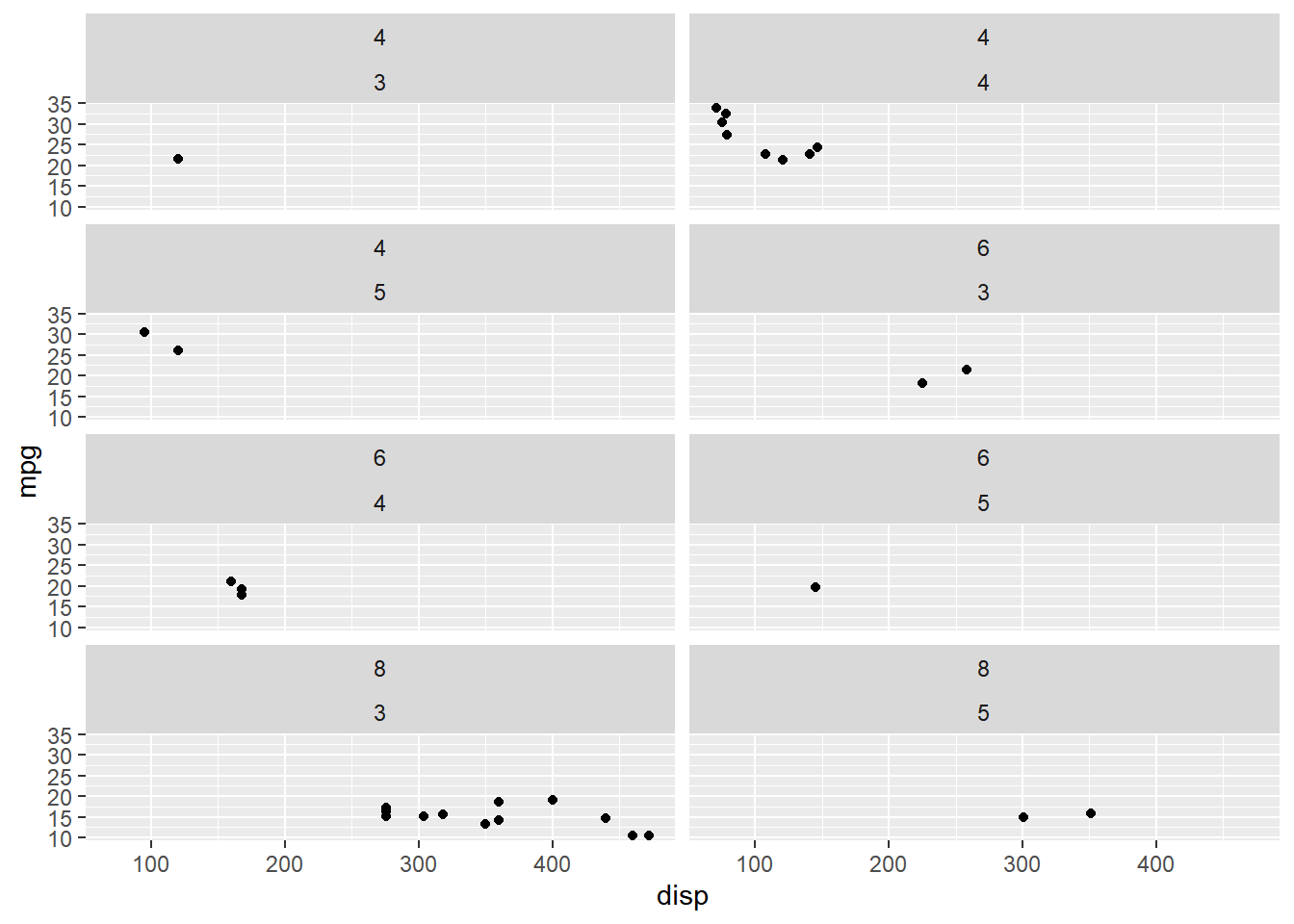### Summary

In this post, we learnt to create sub plots using:

• `facet_grid()`
• `facet_wrap()`

### Up Next..

In the next post, we will learn to modify the theme of a plot.